# tfestimate

Transfer function estimate

## Syntax

``txy = tfestimate(x,y)``
``txy = tfestimate(x,y,window)``
``txy = tfestimate(x,y,window,noverlap)``
``txy = tfestimate(x,y,window,noverlap,nfft)``
``txy = tfestimate(___,'mimo')``
``[txy,w] = tfestimate(___)``
``[txy,f] = tfestimate(___,fs)``
``[txy,w] = tfestimate(x,y,window,noverlap,w)``
``[txy,f] = tfestimate(x,y,window,noverlap,f,fs)``
``[___] = tfestimate(x,y,___,freqrange)``
``[___] = tfestimate(___,'Estimator',est)``
``tfestimate(___)``

## Description

````txy = tfestimate(x,y)` finds a transfer function estimate between the input signal `x` and the output signal `y` evaluated at a set of frequencies. If `x` and `y` are both vectors, they must have the same length.If one of the signals is a matrix and the other is a vector, then the length of the vector must equal the number of rows in the matrix. The function expands the vector and returns a matrix of column-by-column transfer function estimates.If `x` and `y` are matrices with the same number of rows but different numbers of columns, then `txy` is a multi-input/multi-output (MIMO) transfer function that combines all input and output signals. `txy` is a three-dimensional array. If `x` has m columns and `y` has n columns, then `txy` has n columns and m pages. See Transfer Function for more information.If `x` and `y` are matrices of equal size, then `tfestimate` operates column-wise: ```txy(:,n) = tfestimate(x(:,n),y(:,n))```. To obtain a MIMO estimate, append `'mimo'` to the argument list. ```

example

````txy = tfestimate(x,y,window)` uses `window` to divide `x` and `y` into segments and perform windowing.```
````txy = tfestimate(x,y,window,noverlap)` uses `noverlap` samples of overlap between adjoining segments.```
````txy = tfestimate(x,y,window,noverlap,nfft)` uses `nfft` sampling points to calculate the discrete Fourier transform.```
````txy = tfestimate(___,'mimo')` computes a MIMO transfer function for matrix inputs. This syntax can include any combination of input arguments from previous syntaxes.```
````[txy,w] = tfestimate(___)` returns a vector of normalized frequencies, `w`, at which the transfer function is estimated.```

example

````[txy,f] = tfestimate(___,fs)` returns a vector of frequencies, `f`, expressed in terms of the sample rate, `fs`, at which the transfer function is estimated. `fs` must be the sixth numeric input to `tfestimate`. To input a sample rate and still use the default values of the preceding optional arguments, specify these arguments as empty `[]`.```
````[txy,w] = tfestimate(x,y,window,noverlap,w)` returns the transfer function estimate at the normalized frequencies specified in `w`.```
````[txy,f] = tfestimate(x,y,window,noverlap,f,fs)` returns the transfer function estimate at the frequencies specified in `f`.```
````[___] = tfestimate(x,y,___,freqrange)` returns the transfer function estimate over the frequency range specified by `freqrange`. Valid options for `freqrange` are `'onesided'`, `'twosided'`, and `'centered'`.```

example

````[___] = tfestimate(___,'Estimator',est)` estimates transfer functions using the estimator `est`. Valid options for `est` are `'H1'` and `'H2'`.```
````tfestimate(___)` with no output arguments plots the transfer function estimate in the current figure window.```

## Examples

collapse all

Compute and plot the transfer function estimate between two sequences, `x` and `y`. The sequence `x` consists of white Gaussian noise. `y` results from filtering `x` with a 30th-order lowpass filter with normalized cutoff frequency $0.2\pi$ rad/sample. Use a rectangular window to design the filter. Specify a sample rate of 500 Hz and a Hamming window of length 1024 for the transfer function estimate.

```h = fir1(30,0.2,rectwin(31)); x = randn(16384,1); y = filter(h,1,x); fs = 500; tfestimate(x,y,1024,[],[],fs)```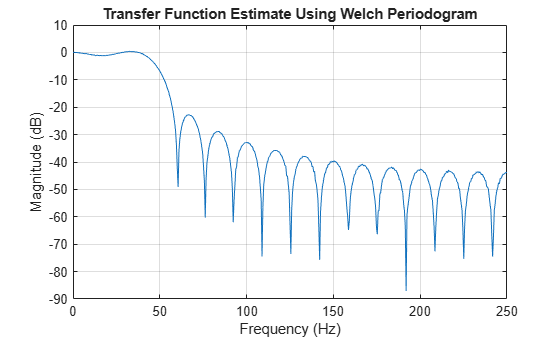Use `fvtool` to verify that the transfer function approximates the frequency response of the filter.

`fvtool(h,1,'Fs',fs)`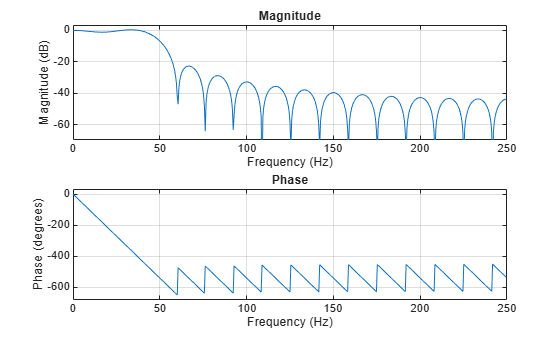Obtain the same result by returning the transfer function estimate in a variable and plotting its absolute value in decibels.

```[Txy,f] = tfestimate(x,y,1024,[],[],fs); plot(f,mag2db(abs(Txy)))```Estimate the transfer function for a simple single-input/single-output system and compare it to the definition.

A one-dimensional discrete-time oscillating system consists of a unit mass, $\mathit{m}$, attached to a wall by a spring of unit elastic constant. A sensor samples the acceleration, $\mathit{a}$, of the mass at ${\mathit{F}}_{\mathit{s}}=1$ Hz. A damper impedes the motion of the mass by exerting on it a force proportional to speed, with damping constant $\mathit{b}=0.01$.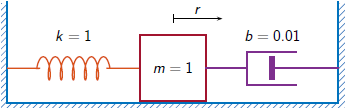Generate 2000 time samples. Define the sampling interval $\Delta \mathit{t}=1/{\mathit{F}}_{\mathit{s}}$.

```Fs = 1; dt = 1/Fs; N = 2000; t = dt*(0:N-1); b = 0.01;```

The system can be described by the state-space model

`$\begin{array}{c}x\left(k+1\right)=Ax\left(k\right)+Bu\left(k\right),\\ y\left(k\right)=Cx\left(k\right)+Du\left(k\right),\end{array}$`

where $\mathit{x}={\left[\begin{array}{cc}\mathit{r}& \mathit{v}\end{array}\right]}^{\mathit{T}}$ is the state vector, $\mathit{r}$ and $\mathit{v}$ are respectively the position and velocity of the mass, $\mathit{u}$ is the driving force, and $\mathit{y}=\mathit{a}$ is the measured output. The state-space matrices are

`$A=\mathrm{exp}\left({A}_{c}\Delta t\right),\phantom{\rule{1em}{0ex}}B={A}_{c}^{-1}\left(A-I\right){B}_{c},\phantom{\rule{1em}{0ex}}C=\left[\begin{array}{cc}-1& -b\end{array}\right],\phantom{\rule{1em}{0ex}}D=1,$`

$\mathit{I}$ is the $2×2$ identity, and the continuous-time state-space matrices are

`${A}_{c}=\left[\begin{array}{cc}0& 1\\ -1& -b\end{array}\right],\phantom{\rule{1em}{0ex}}{B}_{c}=\left[\begin{array}{c}0\\ 1\end{array}\right].$`

```Ac = [0 1;-1 -b]; A = expm(Ac*dt); Bc = [0;1]; B = Ac\(A-eye(size(A)))*Bc; C = [-1 -b]; D = 1;```

The mass is driven by random input for half of the measurement interval. Use the state-space model to compute the time evolution of the system starting from an all-zero initial state. Plot the acceleration of the mass as a function of time.

```rng default u = zeros(1,N); u(1:N/2) = randn(1,N/2); y = 0; x = [0;0]; for k = 1:N y(k) = C*x + D*u(k); x = A*x + B*u(k); end plot(t,y)```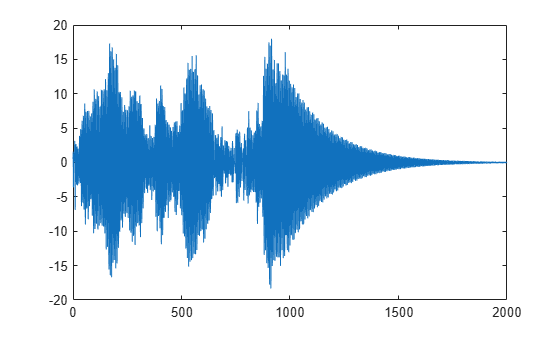Estimate the transfer function of the system as a function of frequency. Use 2048 DFT points and specify a Kaiser window with a shape factor of 15. Use the default value of overlap between adjoining segments.

```nfs = 2048; wind = kaiser(N,15); [txy,ft] = tfestimate(u,y,wind,[],nfs,Fs);```

The frequency-response function of a discrete-time system can be expressed as the Z-transform of the time-domain transfer function of the system, evaluated at the unit circle. Verify that the estimate computed by `tfestimate` coincides with this definition.

```[b,a] = ss2tf(A,B,C,D); fz = 0:1/nfs:1/2-1/nfs; z = exp(2j*pi*fz); frf = polyval(b,z)./polyval(a,z); plot(ft,20*log10(abs(txy))) hold on plot(fz,20*log10(abs(frf))) hold off grid ylim([-60 40])```Plot the estimate using the built-in functionality of `tfestimate`.

`tfestimate(u,y,wind,[],nfs,Fs)`Estimate the transfer function for a simple multi-input/multi-output system.

An ideal one-dimensional oscillating system consists of two masses, ${\mathit{m}}_{1}$ and ${\mathit{m}}_{2}$, confined between two walls. The units are such that ${\mathit{m}}_{1}=1$ and ${\mathit{m}}_{2}=\mu$. Each mass is attached to the nearest wall by a spring with an elastic constant $\mathit{k}$. An identical spring connects the two masses. Three dampers impede the motion of the masses by exerting on them forces proportional to speed, with damping constant $\mathit{b}$. Sensors sample ${\mathit{a}}_{1}$ and ${\mathit{a}}_{2}$, the accelerations of the masses, at ${\mathit{F}}_{\mathit{s}}=50$ Hz.Generate 30000 time samples, equivalent to 600 seconds. Define the sampling interval $\Delta \mathit{t}=1/{\mathit{F}}_{\mathit{s}}$.

```Fs = 50; dt = 1/Fs; N = 30000; t = dt*(0:N-1);```

The system can be described by the state-space model

`$\begin{array}{c}x\left(k+1\right)=Ax\left(k\right)+Bu\left(k\right),\\ y\left(k\right)=Cx\left(k\right)+Du\left(k\right),\end{array}$`

where $x={\left[\begin{array}{cccc}{r}_{1}& {v}_{1}& {r}_{2}& {v}_{2}\end{array}\right]}^{T}$ is the state vector, ${r}_{i}$ and ${v}_{i}$ are respectively the location and the velocity of the $i$th mass, $u={\left[\begin{array}{cc}{u}_{1}& {u}_{2}\end{array}\right]}^{T}$ is the vector of input driving forces, and $y={\left[\begin{array}{cc}{a}_{1}& {a}_{2}\end{array}\right]}^{T}$ is the output vector. The state-space matrices are

`$A=\mathrm{exp}\left({A}_{c}\Delta t\right),\phantom{\rule{1em}{0ex}}B={A}_{c}^{-1}\left(A-I\right){B}_{c},\phantom{\rule{1em}{0ex}}C=\left[\begin{array}{cccc}-2k& -2b& k& b\\ k/\mu & b/\mu & -2k/\mu & -2b/\mu \end{array}\right],\phantom{\rule{1em}{0ex}}D=\left[\begin{array}{cc}1& 0\\ 0& 1/\mu \end{array}\right],$`

$\mathit{I}$ is the $4×4$ identity, and the continuous-time state-space matrices are

`${A}_{c}=\left[\begin{array}{cccc}0& 1& 0& 0\\ -2k& -2b& k& b\\ 0& 0& 0& 1\\ k/\mu & b/\mu & -2k/\mu & -2b/\mu \end{array}\right],\phantom{\rule{1em}{0ex}}{B}_{c}=\left[\begin{array}{cc}0& 0\\ 1& 0\\ 0& 0\\ 0& 1/\mu \end{array}\right].$`

Set $\mathit{k}=400$, $\mathit{b}=0$, and $\mu =1/10$.

```k = 400; b = 0; m = 1/10; Ac = [0 1 0 0;-2*k -2*b k b;0 0 0 1;k/m b/m -2*k/m -2*b/m]; A = expm(Ac*dt); Bc = [0 0;1 0;0 0;0 1/m]; B = Ac\(A-eye(4))*Bc; C = [-2*k -2*b k b;k/m b/m -2*k/m -2*b/m]; D = [1 0;0 1/m];```

The masses are driven by random input throughout the measurement. Use the state-space model to compute the time evolution of the system starting from an all-zero initial state.

```rng default u = randn(2,N); x = [0;0;0;0]; for kk = 1:N y(:,kk) = C*x + D*u(:,kk); x = A*x + B*u(:,kk); end```

Use the input and output data to estimate the transfer function of the system as a function of frequency. Specify the `'mimo'` option to produce all four transfer functions. Use a 5000-sample Hann window to divide the signals into segments. Specify 2500 samples of overlap between adjoining segments and ${2}^{14}$ DFT points. Plot the estimates.

```wind = hann(5000); nov = 2500; [q,fq] = tfestimate(u',y',wind,nov,2^14,Fs,'mimo');```

Compute the theoretical transfer function as the Z-transform of the time-domain transfer function, evaluated at the unit circle.

```nfs = 2^14; fz = 0:1/nfs:1/2-1/nfs; z = exp(2j*pi*fz); [b1,a1] = ss2tf(A,B,C,D,1); [b2,a2] = ss2tf(A,B,C,D,2); frf(1,:,1) = polyval(b1(1,:),z)./polyval(a1,z); frf(1,:,2) = polyval(b1(2,:),z)./polyval(a1,z); frf(2,:,1) = polyval(b2(1,:),z)./polyval(a2,z); frf(2,:,2) = polyval(b2(2,:),z)./polyval(a2,z);```

Plot the theoretical transfer functions and their corresponding estimates.

```for jk = 1:2 for kj = 1:2 subplot(2,2,2*(jk-1)+kj) plot(fq,20*log10(abs(q(:,jk,kj)))) hold on plot(fz*Fs,20*log10(abs(frf(jk,:,kj)))) hold off grid title(['Input ' int2str(kj) ', Output ' int2str(jk)]) axis([0 Fs/2 -50 100]) end end```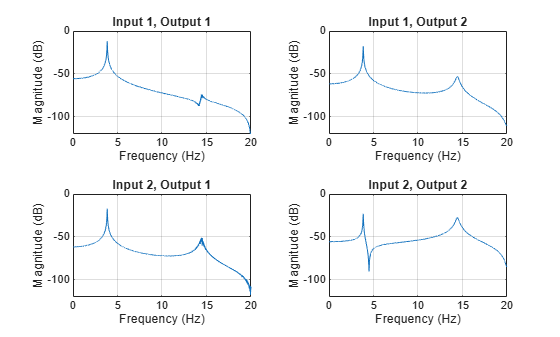The transfer functions have maxima at the expected values, ${\omega }_{1,2}/2\pi$, where the $\omega$ are the eigenvalues of the modal matrix.

`sqrt(eig(k*[2 -1;-1/m 2/m]))/(2*pi)`
```ans = 2×1 3.8470 14.4259 ```

Add damping to the system by setting $\mathit{b}=0.1$. Compute the time evolution of the damped system with the same driving forces. Compute the ${\mathit{H}}_{2}$ estimate of the MIMO transfer function using the same window and overlap. Plot the estimates using the `tfestimate` functionality.

```b = 0.1; Ac = [0 1 0 0;-2*k -2*b k b;0 0 0 1;k/m b/m -2*k/m -2*b/m]; A = expm(Ac*dt); B = Ac\(A-eye(4))*Bc; C = [-2*k -2*b k b;k/m b/m -2*k/m -2*b/m]; x = [0;0;0;0]; for kk = 1:N y(:,kk) = C*x + D*u(:,kk); x = A*x + B*u(:,kk); end clf tfestimate(u',y',wind,nov,[],Fs,'mimo','Estimator','H2') legend('I1, O1','I1, O2','I2, O1','I2, O2')```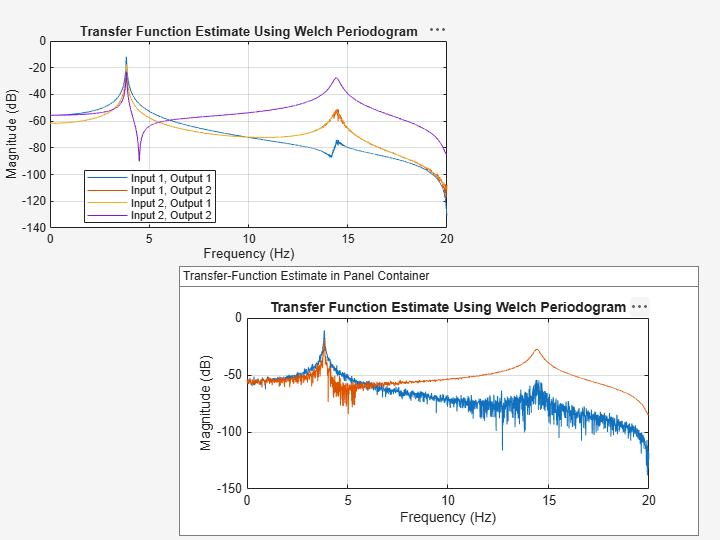`yl = ylim;`

Compare the estimates to the theoretical predictions.

```[b1,a1] = ss2tf(A,B,C,D,1); [b2,a2] = ss2tf(A,B,C,D,2); frf(1,:,1) = polyval(b1(1,:),z)./polyval(a1,z); frf(1,:,2) = polyval(b1(2,:),z)./polyval(a1,z); frf(2,:,1) = polyval(b2(1,:),z)./polyval(a2,z); frf(2,:,2) = polyval(b2(2,:),z)./polyval(a2,z); plot(fz*Fs,20*log10(abs(reshape(permute(frf,[2 1 3]),[nfs/2 4])))) legend('I1, O1','I1, O2','I2, O1','I2, O2') ylim(yl) grid```## Input Arguments

collapse all

Input signal, specified as a vector or matrix.

Example: `cos(pi/4*(0:159))+randn(1,160)` specifies a sinusoid embedded in white Gaussian noise.

Data Types: `single` | `double`
Complex Number Support: Yes

Output signal, specified as a vector or matrix.

Data Types: `single` | `double`
Complex Number Support: Yes

Window, specified as an integer or as a row or column vector. Use `window` to divide the signal into segments.

• If `window` is an integer, then `tfestimate` divides `x` and `y` into segments of length `window` and windows each segment with a Hamming window of that length.

• If `window` is a vector, then `tfestimate` divides `x` and `y` into segments of the same length as the vector and windows each segment using `window`.

If the length of `x` and `y` cannot be divided exactly into an integer number of segments with `noverlap` overlapping samples, then the signals are truncated accordingly.

If you specify `window` as empty, then `tfestimate` uses a Hamming window such that `x` and `y` are divided into eight segments with `noverlap` overlapping samples.

For a list of available windows, see Windows.

Example: `hann(N+1)` and `(1-cos(2*pi*(0:N)'/N))/2` both specify a Hann window of length `N` + 1.

Data Types: `single` | `double`

Number of overlapped samples, specified as a positive integer.

• If `window` is scalar, then `noverlap` must be smaller than `window`.

• If `window` is a vector, then `noverlap` must be smaller than the length of `window`.

If you specify `noverlap` as empty, then `tfestimate` uses a number that produces 50% overlap between segments.

Data Types: `double` | `single`

Number of DFT points, specified as a positive integer. If you specify `nfft` as empty, then `tfestimate` sets this argument to max(256,2p), where p = ⌈log2 N for input signals of length N and the ⌈ ⌉ symbols denote the ceiling function.

Data Types: `single` | `double`

Sample rate, specified as a positive scalar. The sample rate is the number of samples per unit time. If the unit of time is seconds, then the sample rate has units of Hz.

Normalized frequencies, specified as a row or column vector with at least two elements. Normalized frequencies are in rad/sample.

Example: `w = [pi/4 pi/2]`

Data Types: `double`

Frequencies, specified as a row or column vector with at least two elements. The frequencies are in cycles per unit time. The unit time is specified by the sample rate, `fs`. If `fs` has units of samples/second, then `f` has units of Hz.

Example: `fs = 1000; f = [100 200]`

Data Types: `double`

Frequency range for the transfer function estimate, specified as a one of `'onesided'`, `'twosided'`, or `'centered'`. The default is `'onesided'` for real-valued signals and `'twosided'` for complex-valued signals.

• `'onesided'` — Returns the one-sided estimate of the transfer function between two real-valued input signals, `x` and `y`. If `nfft` is even, `txy` has `nfft`/2 + 1 rows and is computed over the interval [0,π] rad/sample. If `nfft` is odd, `txy` has (`nfft` + 1)/2 rows and the interval is [0,π) rad/sample. If you specify `fs`, the corresponding intervals are [0,`fs`/2] cycles/unit time for even `nfft` and [0,`fs`/2) cycles/unit time for odd `nfft`.

• `'twosided'` — Returns the two-sided estimate of the transfer function between two real-valued or complex-valued input signals, `x` and `y`. In this case, `txy` has `nfft` rows and is computed over the interval [0,2π) rad/sample. If you specify `fs`, the interval is [0,`fs`) cycles/unit time.

• `'centered'` — Returns the centered two-sided estimate of the transfer function between two real-valued or complex-valued input signals, `x` and `y`. In this case, `txy` has `nfft` rows and is computed over the interval (–π,π] rad/sample for even `nfft` and (–π,π) rad/sample for odd `nfft`. If you specify `fs`, the corresponding intervals are (–`fs`/2, `fs`/2] cycles/unit time for even `nfft` and (–`fs`/2, `fs`/2) cycles/unit time for odd `nfft`.

Transfer function estimator, specified as `'H1'` or `'H2'`.

• Use `'H1'` when the noise is uncorrelated with the input signals.

• Use `'H2'` when the noise is uncorrelated with the output signals. In this case, the number of input signals must equal the number of output signals.

## Output Arguments

collapse all

Transfer function estimate, returned as a vector, matrix, or three-dimensional array.

Normalized frequencies, returned as a real-valued column vector.

Cyclical frequencies, returned as a real-valued column vector.

collapse all

### Transfer Function

The relationship between the input `x` and output `y` is modeled by the linear, time-invariant transfer function `txy`. In the frequency domain, Y(f) = H(f)X(f).

• For a single-input/single-output system, the H1 estimate of the transfer function is given by

`${H}_{1}\left(f\right)=\frac{{P}_{yx}\left(f\right)}{{P}_{xx}\left(f\right)},$`

where Pyx is the cross power spectral density of `x` and `y`, and Pxx is the power spectral density of `x`. This estimate assumes that the noise is not correlated with the system input.

For multi-input/multi-output (MIMO) systems, the H1 estimator becomes

`${H}_{1}\left(f\right)={P}_{YX}\left(f\right){P}_{XX}^{-1}\left(f\right)=\left[\begin{array}{cccc}{P}_{{y}_{1}{x}_{1}}\left(f\right)& {P}_{{y}_{1}{x}_{2}}\left(f\right)& \cdots & {P}_{{y}_{1}{x}_{m}}\left(f\right)\\ {P}_{{y}_{2}{x}_{1}}\left(f\right)& {P}_{{y}_{2}{x}_{2}}\left(f\right)& \cdots & {P}_{{y}_{2}{x}_{m}}\left(f\right)\\ ⋮& ⋮& \ddots & ⋮\\ {P}_{{y}_{n}{x}_{1}}\left(f\right)& {P}_{{y}_{n}{x}_{2}}\left(f\right)& \cdots & {P}_{{y}_{n}{x}_{m}}\left(f\right)\end{array}\right]\text{\hspace{0.17em}}{\left[\begin{array}{cccc}{P}_{{x}_{1}{x}_{1}}\left(f\right)& {P}_{{x}_{1}{x}_{2}}\left(f\right)& \cdots & {P}_{{x}_{1}{x}_{m}}\left(f\right)\\ {P}_{{x}_{2}{x}_{1}}\left(f\right)& {P}_{{x}_{2}{x}_{2}}\left(f\right)& \cdots & {P}_{{x}_{2}{x}_{m}}\left(f\right)\\ ⋮& ⋮& \ddots & ⋮\\ {P}_{{x}_{m}{x}_{1}}\left(f\right)& {P}_{{x}_{m}{x}_{2}}\left(f\right)& \cdots & {P}_{{x}_{m}{x}_{m}}\left(f\right)\end{array}\right]}^{-1}$`

for m inputs and n outputs, where:

• Pyixk is the cross power spectral density of the kth input and the ith output.

• Pxixk is the cross power spectral density of the kth and ith inputs.

For two inputs and two outputs, the estimator is the matrix

`${H}_{1}\left(f\right)=\frac{\left[\begin{array}{cc}{P}_{{y}_{1}{x}_{1}}\left(f\right){P}_{{x}_{2}{x}_{2}}\left(f\right)-{P}_{{y}_{1}{x}_{2}}\left(f\right){P}_{{x}_{2}{x}_{1}}\left(f\right)& {P}_{{y}_{1}{x}_{2}}\left(f\right){P}_{{x}_{1}{x}_{1}}\left(f\right)-{P}_{{y}_{1}{x}_{1}}\left(f\right){P}_{{x}_{1}{x}_{2}}\left(f\right)\\ {P}_{{y}_{2}{x}_{1}}\left(f\right){P}_{{x}_{2}{x}_{2}}\left(f\right)-{P}_{{y}_{2}{x}_{2}}\left(f\right){P}_{{x}_{2}{x}_{1}}\left(f\right)& {P}_{{y}_{2}{x}_{2}}\left(f\right){P}_{{x}_{1}{x}_{1}}\left(f\right)-{P}_{{y}_{2}{x}_{1}}\left(f\right){P}_{{x}_{1}{x}_{2}}\left(f\right)\end{array}\right]}{{P}_{{x}_{1}{x}_{1}}\left(f\right){P}_{{x}_{2}{x}_{2}}\left(f\right)-{P}_{{x}_{1}{x}_{2}}\left(f\right){P}_{{x}_{2}{x}_{1}}\left(f\right)}.$`

• For a single-input/single-output system, the H2 estimate of the transfer function is given by

`${H}_{2}\left(f\right)=\frac{{P}_{yy}\left(f\right)}{{P}_{xy}\left(f\right)},$`

where Pyy is the power spectral density of `y` and Pxy = P*yx is the complex conjugate of the cross power spectral density of `x` and `y`. This estimate assumes that the noise is not correlated with the system output.

For MIMO systems, the H2 estimator is well-defined only for equal numbers of inputs and outputs: n = m. The estimator becomes

`${H}_{2}\left(f\right)={P}_{YY}\left(f\right){P}_{XY}^{-1}\left(f\right)=\left[\begin{array}{cccc}{P}_{{y}_{1}{y}_{1}}\left(f\right)& {P}_{{y}_{1}{y}_{2}}\left(f\right)& \cdots & {P}_{{y}_{1}{y}_{n}}\left(f\right)\\ {P}_{{y}_{2}{y}_{1}}\left(f\right)& {P}_{{y}_{2}{y}_{2}}\left(f\right)& \cdots & {P}_{{y}_{2}{y}_{n}}\left(f\right)\\ ⋮& ⋮& \ddots & ⋮\\ {P}_{{y}_{n}{y}_{1}}\left(f\right)& {P}_{{y}_{n}{y}_{2}}\left(f\right)& \cdots & {P}_{{y}_{n}{y}_{n}}\left(f\right)\end{array}\right]\text{\hspace{0.17em}}{\left[\begin{array}{cccc}{P}_{{x}_{1}{y}_{1}}\left(f\right)& {P}_{{x}_{1}{y}_{2}}\left(f\right)& \cdots & {P}_{{x}_{1}{y}_{n}}\left(f\right)\\ {P}_{{x}_{2}{y}_{1}}\left(f\right)& {P}_{{x}_{2}{y}_{2}}\left(f\right)& \cdots & {P}_{{x}_{2}{y}_{n}}\left(f\right)\\ ⋮& ⋮& \ddots & ⋮\\ {P}_{{x}_{n}{y}_{1}}\left(f\right)& {P}_{{x}_{n}{y}_{2}}\left(f\right)& \cdots & {P}_{{x}_{n}{y}_{n}}\left(f\right)\end{array}\right]}^{-1},$`

where:

• Pyiyk is the cross power spectral density of the kth and ith outputs.

• Pxiyk is the complex conjugate of the cross power spectral density of the ith input and the kth output.

## Algorithms

`tfestimate` uses Welch's averaged periodogram method. See `pwelch` for details.

 Vold, Håvard, John Crowley, and G. Thomas Rocklin. “New Ways of Estimating Frequency Response Functions.” Sound and Vibration. Vol. 18, November 1984, pp. 34–38.